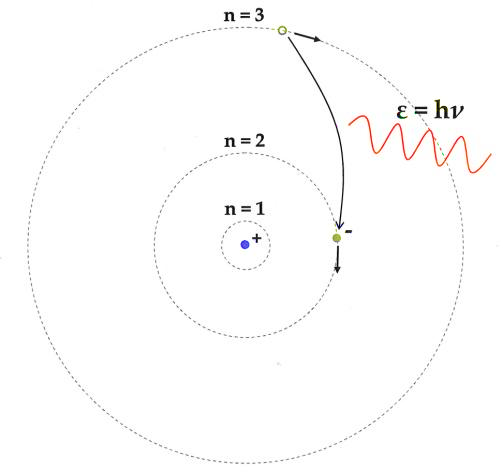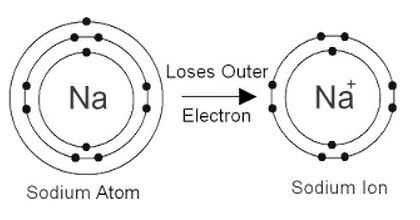Courses
Courses for Kids
Free study material
Offline Centres
MoreLast updated date: 04th Dec 2023
Total views: 280.2k
Views today: 4.80k

# What are the postulates of Bohr's model of an atom? Show diagrammatically the electron distribution in a sodium atom and a sodium ion and also give their atomic number.Verified
280.2k+ views
Hint :
We must note that Neil Bohr presented the Bohr model of the atom in 1915. With the alteration of Rutherford's atomic model, it became a possibility. According to Rutherford's model, a positively charged nucleus is surrounded by negatively charged electrons. Bohr changed the atomic structure model by stating that electrons are confined to fixed orbitals known as shells. Each orbit, he explained, has a set energy level. Rutherford identified an atom's nucleus, which Bohr modified into electrons and their energy levels.

The following are the postulates of Bohr's atomic model:
1. Negatively charged electrons in an atom rotate around the positively charged nucleus in a spiral direction known as orbits or shells.
2. The circular orbits are known as orbital shells because each orbit or shell has a set energy.
3. The quantum number is an integer n =1,2,3,... that denotes the energy levels. This collection of quantum numbers begins on the nucleus's side, with n=1 being the lowest energy density. The orbits n=1,2,3,4 are known as K, L, M, N.... shells, and an electron is assumed to be in the ground state when it approaches the lowest energy level.
4. Electrons in an atom gain energy as they move from a lower energy level to a higher energy level, and they lose energy when they go from a higher energy level to a lower energy level.